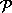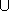## CGAL::surface_neighbor_coordinates_3

### Definition

The function surface_neighbor_coordinates_3 computes natural neighbor coordinates for surface points associated to a finite set of sample points issued from the surface. The coordinates are computed from the intersection of the Voronoi cell of the query point p with the tangent plane to the surface at p. If the sampling is sufficiently dense, the coordinate system meets the properties described in the manual pages and in [BF02],[Flö03]. The query point p needs to lie inside the convex hull of the projection of the sample points onto the tangent plane at p.

#include <CGAL/surface_neighbor_coordinates_3.h>

template <class OutputIterator, class InputIterator, class Kernel>
CGAL::Triple< OutputIterator, typename Kernel::FT, bool >
 surface_neighbor_coordinates_3 ( InputIterator first, InputIterator beyond, typename Kernel::Point_3 p, typename Kernel::Vector_3 normal, OutputIterator out, Kernel K)
The sample pointsare provided in the range [.first, beyond.). InputIterator::value_type is the point type Kernel::Point_3. The tangent plane is defined by the point p and the vector normal. The parameter K determines the kernel type that will instantiate the template parameter of Voronoi_intersection_2_traits_3<K>.
The natural neighbor coordinates for p are computed in the power diagram that results from the intersection of the 3D Voronoi diagram ofwith the tangent plane. The sequence of point/coordinate pairs that is computed by the function is placed starting at out. The function returns a triple with an iterator that is placed past-the-end of the resulting sequence of point/coordinate pairs, the normalization factor of the coordinates and a boolean value which is set to true iff the coordinate computation was successful, i.e. if p lies inside the convex hull of the projection of the pointsonto the tangent plane.

template <class OutputIterator, class InputIterator, class ITraits>
CGAL::Triple< OutputIterator, typename ITraits::FT, bool >
 surface_neighbor_coordinates_3 ( InputIterator first, InputIterator beyond, typename ITraits::Point_2 p, OutputIterator out, ITraits traits)
the same as above only that the traits class must be instantiated by the user. ITraits must be equivalent to Voronoi_intersection_2_traits_3<K>.

The next functions return, in addition, a second boolean value (the fourth value of the quadrupel) that certifies whether or not, the Voronoi cell of p can be affected by points that lie outside the input range, i.e. outside the ball centered on p passing through the furthest sample point from p in the range [.first, beyond.). If the sample points are collected by a k-nearest neighbor or a range search query, this permits to check whether the neighborhood which has been considered is large enough.

template <class OutputIterator, class InputIterator, class Kernel>
CGAL::Quadruple< OutputIterator, typename Kernel::FT, bool, bool >
 surface_neighbor_coordinates_certified_3 ( InputIterator first, InputIterator beyond, typename Kernel::Point_3 p, typename Kernel::Vector_3 normal, OutputIterator out, Kernel K)
Similar to the first function. The additional fourth return value is true if the furthest point in the range [.first, beyond.) is further away from p than twice the distance from p to the furthest vertex of the intersection of the Voronoi cell of p with the tangent plane defined by (p,normal). It is false otherwise.

template <class OutputIterator, class InputIterator, class Kernel>
CGAL::Quadruple< OutputIterator, typename Kernel::FT, bool, bool >
 surface_neighbor_coordinates_certified_3 ( InputIterator first, InputIterator beyond, typename Kernel::Point_2 p, typename Kernel::FT max_distance, OutputIterator out, Kernel kernel)
The same as above except that this function takes the maximal distance from p to the points in the range [.first, beyond.) as additional parameter.

template <class OutputIterator, class InputIterator, class ITraits>
CGAL::Quadruple< OutputIterator, typename ITraits::FT, bool, bool >
 surface_neighbor_coordinates_certified_3 ( InputIterator first, InputIterator beyond, typename ITraits::Point_2 p, OutputIterator out, ITraits traits)
The same as above only that the traits class must be instantiated by the user and without the parameter max_distance. ITraits must be equivalent to Voronoi_intersection_2_traits_3<K>.

template <class OutputIterator, class InputIterator, class ITraits>
CGAL::Quadruple< OutputIterator, typename ITraits::FT, bool, bool >
 surface_neighbor_coordinates_certified_3 ( InputIterator first, InputIterator beyond, typename ITraits::Point_2 p, typename ITraits::FT max_distance, OutputIterator out, ITraits traits)
The same as above with the parameter max_distance.

The next function allows to filter some potential neighbors of the query point p fromvia its three-dimensional Delaunay triangulation. All surface neighbors of p are necessarily neighbors in the Delaunay triangulation of{p}.

template < class Dt, class OutputIterator >
CGAL::Triple< OutputIterator, typename Dt::Geom_traits::FT, bool >
 surface_neighbor_coordinates_3 ( Dt dt, typename Dt::Geom_traits::Point_2 p, typename Dt::Geom_traits::Vector_3 normal, OutputIterator out, typename Dt::Face_handle start = typename Dt::Face_handle())
computes the surface neighbor coordinates with respect to the points that are vertices of the Delaunay triangulation dt. The type Dt must be equivalent to Delaunay_triangulation_3<Gt, Tds>. The optional parameter start is used as a starting place for the search of the conflict zone. It may be the result of the call dt.locate(p). This function instantiates the template parameter ITraits to be Voronoi_intersection_2_traits_3<Dt::Geom_traits>.

template < class Dt, class OutputIterator, class ITraits>
CGAL::Triple< OutputIterator, typename Dt::Geom_traits::FT, bool >
 surface_neighbor_coordinates_3 ( Dt dt, typename Dt::Geom_traits::Point_2 p, OutputIterator out, ITraits traits, typename Dt::Face_handle start = typename Dt::Face_handle())
The same as above only that the parameter traits instantiates the geometric traits class. Its type ITraits must be equivalent to Voronoi_intersection_2_traits_3<K>.

### Requirements

1. Dt is equivalent to the class Delaunay_triangulation_3.
2. OutputIterator::value_type is equivalent to std::pair<Dt::Point_3, Dt::Geom_traits::FT>, i.e. a pair associating a point and its natural neighbor coordinate.
3. ITraits is equivalent to the class Voronoi_intersection_2_traits_3<K>.